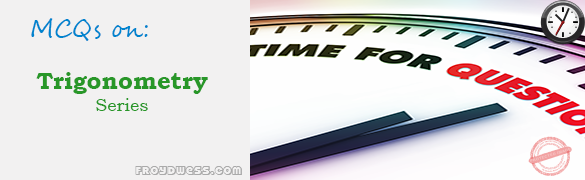# MCQs in Trigonometry Part II

(Last Updated On: December 8, 2017)This is the Multiple Choice Questions Part 2 of the Series in Trigonometry topic in Engineering Mathematics. In Preparation for the ECE Board Exam make sure to expose yourself and familiarize in each and every questions compiled here taken from various sources including but not limited to past Board Examination Questions in Engineering Mathematics, Mathematics Books, Journals and other Mathematics References.

### Multiple Choice Questions Topic Outline

• MCQs in Logarithmic Principles | MCQs in Trigonometric Functions | MCQs in Fundamental Trigonometric Identities | MCQs in Solution of Right and Oblique Triangles | MCQs in Applications of Terrestrial Mensuration | MCQs in Area, Perimeter and Centroid of Plane Figures | MCQs in Polar Coordinates | MCQs in Spherical Trigonometry

### Online Questions and Answers in Trigonometry Series

Following is the list of multiple choice questions in this brand new series:

Trigonometry MCQs
PART 1: MCQs from Number 1 – 50                        Answer key: PART I
PART 2: MCQs from Number 51 – 100                        Answer key: PART II

### Continue Practice Exam Test Questions Part II of the Series

Choose the letter of the best answer in each questions.

51. Solve for side b of a right spherical triangle ABC whose parts are a = 46°, c = 75° and C = 90°.

• A. 74°
• B. 68°
• C. 48°
• D. 74°

52. Given a right spherical triangle whose parts are a = 82°, b = 62°, and C = 90°. What is the value of the side opposite the right angle?

• A. 83°30’
• B. 84°45’
• C. 86°15’
• D. 85°15’

53. Determine the value of the angle of an isosceles spherical triangle ABC whose given parts are b = c = 54°28’ and a = 92°30’.

• A. 89°45’
• B. 55°45’
• C. 84°25’
• D. 41°45’

54. Solve the angle A in the spherical triangle ABC given a = 106°25’, c = 42°16’ and B = 114°53’

• A. 45°54’
• B. 80°42’
• C. 97°09’
• D. 72°43’

55. Solve for angle C of the oblique spherical triangle ABC given, a = 80°, c = 115° and A = 72°

• A. 61°
• B. 85°
• C. 95°
• D. 119°

56. Determine the spherical excess of the spherical triangle ABC given a = 56°, b = 65°, and c = 78°.

• A. 33°33’
• B. 68°37’
• C. 90°57’
• D. 98°45’

57. What is the spherical excess of a spherical triangle whose angles are all right angles?

• A. 45°
• B. 90°
• C. 60°
• D. 30°

58. The area of a spherical triangle ABC whose parts are A = 93°40’, B = 64°12’, C = 116°51’ and the radius if the sphere is 100 m is:

• A. 15613 sq. m
• B. 16531 sq. m
• C. 18645 sq. m
• D. 25612 sq. m

59. A spherical triangle has an area of 327.25 sq. km. What is the radius of the sphere if its spherical excess is 30°?

• A. 20 km
• B. 22 km
• C. 25 km
• D. 28 km

60. A ship on a certain day is at latitude 20°N and longitude 140°E. After sailing for 150 hours at a uniform speed along a great circle route, it reaches a point at latitude 10oS and longitude 170°E. If the radius of the earth is 3959 miles, find the speed in miles per hour.

• A. 174.
• B. 15.4
• C. 16.4
• D. 19.4

61. Sin ( B – A ) is equal to ____________, when B = 270 degrees and A is an acute angle.

• a. –cos A
• b. cos A
• c. –sin A
• d. sin A

62. If sec^2 (A) is 5/2, the quantity 1-sin^2 (A) is equivalent to

• a. 2.5
• b. 1.5
• c. 0.4
• d. 0.6

63. (cos A)^4 – (sin A)^4 is equal to _____

• a. cos 4A
• b. cos 2A
• c. sin 2A
• d. sin 4A

64. Of what quadrant is A, if sec A is positive and csc A is negative?

• a. IV
• b. II
• c. III
• d. I

65. Angles are measured from the positive horizontal axis, and the positive direction is counterclockwise. What are the values of sin B and cos B in the 4th quadrant?

• a. sin B > 0 and cos B < 0
• b. sin B < 0 and cos B < 0
• c. sin B > 0 and cos B > 0
• d. sin B < 0 and cos B > 0
MCQs in Trigonometry Part II
3 (60%) 1 vote